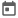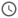# Verilog Assignments##### 09 Sep 2021##### 6 mins

In Verilog, there are various ways for assignment, due to the concurrent nature of the Verilog code. Also, to represent the combinational and sequential digital circuits, Verilog provides different ways for assignment which helps to model the hardware accurately.

As we know, Verilog has `net` and `reg` data types, which represent a `wire` and `flip flop` respectively. From hardware point of view, wires are driven continuously once the circuit is switched on, thus for every point of time wire will take the value which is fed into as it cannot retain any previous value. To represent this behaviour, Verilog provides continuous assignment. This will assign certain value to the wire at every time step.

Similarly, flip flops, are not driven continuously, rather it is driven at some clock edge or any other event, as flip flops retain the value until it is changed. This is the expected behaviour in sequential circuits. To represent this behaviour, Verilog provides procedural assignment, in which the assignment will be done only if certain event is triggered. Let’s see these assignments in detail.

### Continuous Assignment

As discussed earlier this assignment is generally used for `net` data types. `assign` keyword is used for continuous assignment and is used directly inside the module, i.e., procedural blocks are not required for this type of assignment.

### Procedural Assignment

Procedural assignments are used with flip flops, i.e., for sequential circuits. Thus, it can be used to drive only variables and not any `net` data type. Also, this type of assignment can only be used inside a procedural block, i.e., `initial` or `always`.

Procedural assignment can further be divided into 2 types:

1. Blocking Assignment
2. Non-blocking Assignments

#### Blocking Assignment

This type of assignment is the same as we see in all the programming language. As the name suggests, program flow will be blocked until the assignment is complete. This assignment is done using the help of `=` operator, which is also known as blocking assignment operator. Blocking assignment is executed in the Active region of the event semantics. As we know, the active region does not guarantee the order of execution, thus this type of assignment is prone to race conditions as discussed in previous article.

#### Non-blocking Assignment

This type of assignment, as name suggests, does not block the flow of program. The RHS of the assignment operation is calculated but it is not assigned to LHS. All the non-blocking assignments are executed at the end of the time-step in NBA region of event semantics and the LHS gets assigned with the calculated RHS. NBAs are done using `<=` operator which is also known as non-blocking assignment operator. As this assignment is done in NBA region, it helps prevent race around condition. We will see how this prevents race around condition with example later in this article.

``````module assignment_demo;
reg [3:0] a;
wire [3:0] b;

// continous assignment
assign b = a;
// assign a = 4'd6; // Not Valid

// continous assignment
initial begin
\$monitor("At [%0t], value of a = %0d & b = %0d",\$time, a, b);
a = 4'd3;
#10 a = 4'd8; // blocking assignment
#10 a <= 4'd2;// non blocking assignment
// b = 4'ds8; // Not Valid
end
endmodule``````

### Procedural Continuous Assignment

This is a continuous assignment which is used inside the procedural blocks. This is mainly used when we want to override the value of a variable or net. These types of assignments can be achieved using

1. `assign` - `deassign` keyword
2. `force` - `release` keyword.

#### Assign deassign keywords

These are used to override the value of a variable until the variable is de-assigned using `deassign` keyword. After de-assignment, the value of the variable will remain the same until it is re-assigned using procedural or procedural continuous assignment.
These can be used only used when LHS is a variable or concatenation of variable.

In below example, the value of `a` is continuously incremented in the first initial block. In the second initial block, at t=17, the value of a is overridden using `assign` keyword, and thus the value of `a` is not getting incremented. Once `deassign` is used at t=27, the value of `a` starts getting incremented.

``````module assign_deassign;
reg [3:0] a;

initial begin
a = 4'd0;
\$monitor("At [%0t], value of a = %0d", \$time, a);
repeat(10) begin
\$display("Incrementing a");
a = a + 1;
// \$display("At [%0t], value of a = %0d", \$time, a);
#5;
end
end

initial begin
#17;
\$display("At [%0t], Overriding a with assign", \$time);
assign a = 4'he;
#10
\$display("At [%0t], Deassigning a", \$time);
deassign a;
end
endmodule``````
##### Output

The value of `a` is not getting printed once the value of `a` is overridden, as `\$monitor` prints only when the value of the variable changes. As assign does not let the value change, thus value of `a` is not getting printed even after `a` is incremented.

``````# Incrementing a
# At , value of a = 1
# Incrementing a
# At , value of a = 2
# Incrementing a
# At , value of a = 3
# Incrementing a
# At , value of a = 4
# At , Overriding a with assign
# At , value of a = 14
# Incrementing a
# Incrementing a
# At , Deassigning a
# Incrementing a
# At , value of a = 15
# Incrementing a
# At , value of a = 0
# Incrementing a
# At , value of a = 1
# Incrementing a
# At , value of a = 2``````

#### Force release keyword

These are same as that of `assign-deassign` statement but it can be used for both nets and variables. The LHS can be a bit-select, part-select of net but cannot be an array or a bit or part select of variables. These will override all other assignments until released.

In below example, `b` is continuously assigned `~a`, i.e., inverse of a. In first initial block value of `a` is incremented and the value of `b` also changes. In second initial block, at t=15 value of a is overridden using `force` keyword. Value of `b` changes with response to `a`. At t=25 value of `b[2:1]` is overridden with `force` keyword, and thus now only the first and last bit of b can change. At t=35 variable `a` is released, and value of a can be changed, but net `b` is still not released, so only the first and the last bit of `b` changes with change in `a`. At t=45 net `b` is also released and now all bits of `b` is changed with change in `a`.

``````module force_release;
wire [3:0] b;
reg [3:0] a;

assign b = ~a;

initial begin
a = 0;
\$monitor("At [%0t], a = %b, b = %b", \$time, a, b);
repeat(12) begin
a = a + 1;
#5;
end
end

initial begin
#15;
\$display("Overriding value of variable a by force");
force a = 4'b1111;
#10;
\$display("Overriding value of part-select of net b by force");
force b[2:1] = 2'b10;
#10
\$display("Releasing a");
release a;
#10
\$display("Releasing b");
release b;
end
endmodule``````
##### Output
``````# At , a = 0001, b = 1110
# At , a = 0010, b = 1101
# At , a = 0011, b = 1100
# Overriding value of variable a by force
# At , a = 1111, b = 0000
# Overriding value of part-select of net b by force
# At , a = 1111, b = 0100
# Releasing a
# At , a = 0000, b = 1101
# At , a = 0001, b = 1100
# Releasing b
# At , a = 0010, b = 1101
# At , a = 0011, b = 1100
# At , a = 0100, b = 1011``````

### Prevention of race around condition

Race around condition, which was discussed in earlier article, can be prevented by using a non-blocking assignment. As we know in non-blocking assignment, the LHS is assigned in the non-blocking region of event semantics, which comes after the active regions, thus the value is determinate as all the calculations have been already done. Let’s understand this with an example.

#### Example

In the 1st code, at the positive edge of `clk`, variable `a` is assigned a value whereas at the same time `b` is reading of value of `a`. As order of execution in active region is not guaranteed in Verilog, thus it can lead to a race around condition.

Whereas in 2nd code, as non-blocking assignment is used, thus `1` will not be assigned immediately to `a`. Now when, `b` access the variable `a` it will always read the previous value stored, in this case `0`. Thus, `b` will be assigned with `0` and `a` will be assigned with `1` at the send of the time step. Also note that for `b` to attain the value of `a`, it takes 2 cycles, thus at t=30, `b` = 1

##### 1st code - having race around condition
``````module race_around;
reg a;
reg b;
reg clk;

initial begin
a = 0;
b = 0;
clk = 0;
\$monitor("At [%0t] a = %b, b = %b", \$time,a ,b);
#50 \$finish;
end

always #10 clk = ~clk;

always @(posedge clk) begin
a = 1;
end

always @(posedge clk) begin
b = a;
end
endmodule``````
##### Output
``````# At  a = 0, b = 0
# At  a = 1, b = 1``````
Try this code in EDA Playground
##### 2nd code - solution for race condition
``````module race_around_sol;
reg a;
reg b;
reg clk;

initial begin
a = 0;
b = 0;
clk = 0;
\$monitor("At [%0t] a = %b, b = %b", \$time,a ,b);
#50 \$finish;
end

always #10 clk = ~clk;

always @(posedge clk) begin
a <= 1;
end

always @(posedge clk) begin
b <= a;
end
endmodule``````
##### Output
``````# At  a = 0, b = 0
# At  a = 1, b = 0
# At  a = 1, b = 1``````
Try this code in EDA Playground